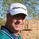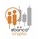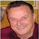35770瀏覽
Enhanced Parabolic Sar

Simply Enhances Default Parabolic SAR by creating Two Color Options, One for UpTrend, Other for DownTrend

Ability To Turn On/Off The Up Trending Parabolic SAR , And The Down Trending Parabolic SAR

Great Indicator For Trailing Stops.
```//Created By ChrisMoody on 7/25/2014
//Simply Enhances Default Parabolic SAR by creating Two Color Options, One for UpTrend, Other for DownTrend
//Ability To Turn On/Off The Up Trending Parabolic SAR, And The Down Trending Parabolic SAR
study(title="CM_Parabolic SAR", shorttitle="CM_P-SAR", overlay=true)
start = input(2, minval=0, maxval=10, title="Start - Default = 2 - Multiplied by .01")
increment = input(2, minval=0, maxval=10, title="Step Setting (Sensitivity) - Default = 2 - Multiplied by .01" )
maximum = input(2, minval=1, maxval=10, title="Maximum Step (Sensitivity) - Default = 2 - Multiplied by .10")
sus = input(true, "Show Up Trending Parabolic Sar")
sds = input(true, "Show Down Trending Parabolic Sar")
disc = input(false, title="Start and Step settings are *.01 so 2 = .02 etc, Maximum Step is *.10 so 2 = .2")
//"------Step Setting Definition------"
//"A higher step moves SAR closer to the price action, which makes a reversal more likely."
//"The indicator will reverse too often if the step is set too high."

//"------Maximum Step Definition-----")
//"The sensitivity of the indicator can also be adjusted using the Maximum Step."
//"While the Maximum Step can influence sensitivity, the Step carries more weight"
//"because it sets the incremental rate-of-increase as the trend develops"

startCalc = start * .01
incrementCalc = increment * .01
maximumCalc = maximum * .10

sarUp = sar(startCalc, incrementCalc, maximumCalc)
sarDown = sar(startCalc, incrementCalc, maximumCalc)

colUp = close >= sarDown ? lime : na
colDown = close <= sarUp ? red : na

plot(sus and sarUp ? sarUp : na, title="Up Trending SAR", style=circles, linewidth=4,color=colUp)
plot(sds and sarDown ? sarDown : na, title="Up Trending SAR", style=circles, linewidth=4,color=colDown)```

## 評論It is possible to have alerts when SAR going Down or Upmthibault1963
@mthibault1963, here is the updated Chris's script with Buy and Sell Alerts!!!

Hope this helps.

//@version=3
start = input(2, minval=0, maxval=10, title="Start - Default = 2 - Multiplied by .01")
increment = input(2, minval=0, maxval=10, title="Step Setting (Sensitivity) - Default = 2 - Multiplied by .01" )
maximum = input(2, minval=1, maxval=10, title="Maximum Step (Sensitivity) - Default = 2 - Multiplied by .10")
sus = input(true, "Show Up Trending Parabolic Sar")
sds = input(true, "Show Down Trending Parabolic Sar")
disc = input(false, title="Start and Step settings are *.01 so 2 = .02 etc, Maximum Step is *.10 so 2 = .2")
highlightStartPoints = input(title="Highlight Start Points ?", defval=true)

startCalc = start * .01
incrementCalc = increment * .01
maximumCalc = maximum * .10

psar = sar(startCalc, incrementCalc, maximumCalc)
dir = psar < close ? 1 : -1

colUp = close >= psar ? lime : na
colDown = close <= psar ? red : na

plotshape(dir == 1 and dir == -1 and highlightStartPoints ? psar : na, title="Buy", style=shape.labelup, location=location.absolute, size=size.normal, text="Buy", transp=0, textcolor = white, color=green, transp=0)
plotshape(dir == -1 and dir == 1 and highlightStartPoints ? psar : na, title="Sell", style=shape.labeldown, location=location.absolute, size=size.normal, text="Sell", transp=0, textcolor = white, color=red, transp=0)

plot(sus and psar ? psar : na, title="Up Trending SAR", style=circles, linewidth=4, color=colUp, transp=0)
plot(sds and psar ? psar : na, title="Down Trending SAR", style=circles, linewidth=4, color=colDown, transp=0)

bgcolor(sus and psar ? colUp : na, transp=90, title="BUY NOW")
bgcolor(sds and psar ? colDown: na, transp=90, title="SELL NOW")

alertcondition(condition=dir == -1 and dir == 1 and highlightStartPoints ? psar : na, title="SAR SELL ALERT", message="SAR SELL ALERT")Hi,
I want to set an alert when the green circle compare on candelstick closing.

How can i set it?

ThanksThe alert has just two up trending option and no down trending option.Chris, great indicator. I did revise the Inputs to Floating Point in order to match up closer with issues I follow.
Thanks,
Bill